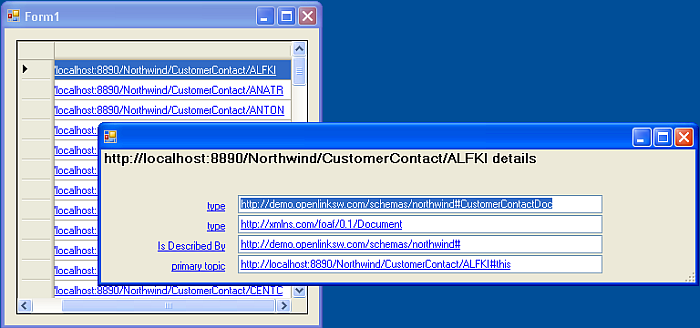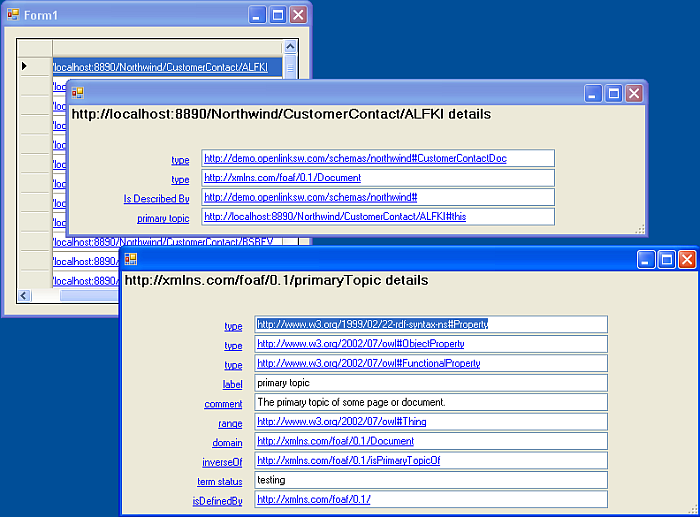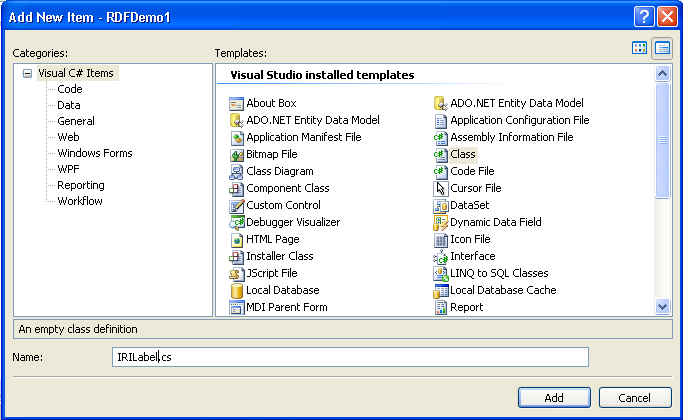### 2.9.7.Extending RDFDemo To Make The Property Labels Clickable

This section will guide you through extending RDFDemo to make the property labels clickable. Clicking on the property label will take you to a page describing that property.

Pre-requisites

1. A working copy of the RDFDemo application created in Extending RDFDemo to Display Images and Longer Text Fields

#### Modifying the Application

Making the Labels Clickable

Making the property labels clickable is fairly straight forward. We will use basically the same code as is used to make the values clickable.

1. First we need to add a new event handler. In the ExtendedStringHandler class modify displayData to add an event handler for each of the property labels.

```for (int i = 0; i < table1.Rows.Count; i++)
{
textBoxList[i].Click += new EventHandler(this.iri_Click);
labelList[i].Location = new Point(10, i * 22 + 50);
textBoxList[i].Location = new Point(150, i * 22 + 50);
```

becomes

```for (int i = 0; i < table1.Rows.Count; i++)
{
textBoxList[i].Click += new EventHandler(this.iri_Click);
labelList[i].Location = new Point(10, i * 22 + 50);
labelList[i].Click += new EventHandler(this.label_Click);
textBoxList[i].Location = new Point(150, i * 22 + 50);
```
2. Then we need to add the EventHandler method. Add the following to the ExtendedStringHandler Class:

```public void label_Click(object sender, EventArgs e)
{
int labelNum = 0;

for (int i = 0; i < labelList.Count; i++)
{
if (sender == labelList[i])
{
labelNum = i;
break;
}
}

Object o = describeDataSet.DataTable1.Rows[labelNum];
if (o is SqlExtendedString)
{
SqlExtendedString se = (SqlExtendedString)o;
ExtendedStringHandler seHandler = new ExtendedStringHandler(se, ParentConnection);
seHandler.displayData();
}
else if (o is SqlRdfBox)
{
//doesn't do anything at the moment
}
}
```

If you compare this method to the EventHandler for the values, iri_Click, you will see that it is basically the same. The only difference is that it uses the property element from the data table rather than value.

3. Finally, to make it clear that the labels are now active links, we will change the label colour to blue and underline them.

``` propertyLabel.Text = getLabelText(row);
propertyLabel.AutoEllipsis = true;
propertyLabel.AutoSize = false;
propertyLabel.Width = 130;
propertyLabel.TextAlign = ContentAlignment.MiddleRight;
```

becomes:

```propertyLabel.Text = getLabelText(row);
propertyLabel.ForeColor = Color.Blue;
propertyLabel.Font = new Font(propertyLabel.Font.FontFamily, propertyLabel.Font.Size, propertyLabel.Font.Style | FontStyle.Underline, propertyLabel.Font.Unit);
propertyLabel.AutoEllipsis = true;
propertyLabel.AutoSize = false;
propertyLabel.Width = 130;
propertyLabel.TextAlign = ContentAlignment.MiddleRight;
```
4. Build and run the application. You will see the familiar starting page. If you then select one of the Customers you will notice the property labels now look like hyperlinks.If you click on one of the label hyperlinks you will see a new form showing detailed information about the property which can itself be explored further by clicking on labels and values.

Figure2.157.labels and valuesThese simple changes work up to a point but are not robust. If you explore the properties used by the local Northwind graph you quickly find that the property details are not found. The application needs some further changes to work consistently. The problem is finding the graph where the property information is defined. The general handler for Extended Strings first checks the local Northwind graph, http://example.com/Northwind, where the Northwind data is held, and then uses the IRI itself as the graph and tries to load that dynamically. This is not working for information about the Northwind properties. These properties are defined in the Northwind ontology, http://demo.openlinksw.com/schemas/northwind. We have already had to find this graph when getting the short label name. We need to be able to associate the property label with the graph where its definition is stored. Then we can search this graph for details about the property when the label is clicked.

Using the Graph Where the Property Label was Found to Find the Property Details

1. Create a new class, IRILabel, that inherits from System.Windows.Forms.Label.

• In the

Solution Explorer

right click on the RDFDemo project and select

then

New Item

.

• Add a new class called IRILabel.cs.```using System.Windows.Forms;
using System.Data;
```

to the using block at the top of the class file. The class definition should look like this:

```class IRILabel : Label
```
3. Paste the following code into the body of the class:

```SqlExtendedString SourceIRI;
String GraphUsed;
VirtuosoConnection ParentConnection;

public IRILabel(Object iri, List<String> graphList, VirtuosoConnection parentConnection)
{
ParentConnection = parentConnection;
if (iri is SqlExtendedString)
{
SourceIRI = (SqlExtendedString)iri;
Text = this.getLabelText(graphList);
}
else
Text = iri.ToString();
}

public SqlExtendedString iri
{
get
{
return SourceIRI;
}
}

public String graph
{
get
{
return GraphUsed;
}
}

private string getLabelText(List<String> graphList)
{
string labelText = SourceIRI.ToString();
Boolean foundLabel = false;
DataSet getLabelDataSet = new DataSet();

//Try finding it in resources graph first
foreach (String graph in graphList)
{
StringBuilder getLabelCommandText = new StringBuilder("sparql select * from <" + graph + "> where {<" + SourceIRI.ToString() + "> ?p ?o}");
VirtuosoCommand getLabelCommand = new VirtuosoCommand(getLabelCommandText.ToString(), ParentConnection);

try
{
foreach (DataRow getLabelRow in getLabelDataSet.Tables.Rows)
{
if (getLabelRow.ToString() == "http://www.w3.org/2000/01/rdf-schema#label")
{
labelText = getLabelRow.ToString();
foundLabel = true;
break;
}
}
}
catch
{
}
if (foundLabel)
{
GraphUsed = graph;
break;
}
}

// If we still have no label try the predicate itself as the graph
if (!foundLabel)
{
GraphUsed = SourceIRI.ToString();
StringBuilder getLabelCommandText = new StringBuilder("sparql define get:soft \"soft\" select * from <" + GraphUsed + "> where {<" + SourceIRI.ToString() + "> ?p ?o}");
VirtuosoCommand getLabelCommand = new VirtuosoCommand(getLabelCommandText.ToString(), ParentConnection);
try
{
foreach (DataRow getLabelRow in getLabelDataSet.Tables.Rows)
{
if (getLabelRow.ToString() == "http://www.w3.org/2000/01/rdf-schema#label")
{
labelText = getLabelRow.ToString();
break;
}
}
}
catch
{
}
}
return labelText;
}
```

Notice that the getLabelText method has been moved into this new class and is now called from the constructor. When the IRILabel is constructed the label text is found using the list of graphs provided to the constructor. The graph containing the label is noted. We need to alter ExtendedStringHandler so that the labels are the new IRILabel type and so that the correct information is supplied to the constructor.

4. Change the labelList member variable declaration so it looks like this:

```List<IRILabel> labelList = new List<IRILabel>();
```
5. Each propertyLabel created must be the new IRILabel type so the line:

```Label propertyLabel = new Label();
```

becomes:

```IRILabel propertyLabel = new IRILabel(row, graphList, ParentConnection);
```
6. As getLabelText is now called by the IRILabel constructor we can remove the line:

```propertyLabel.Text = getLabelText(row);
```

from describeData in ExtendedStringHandler.

7. Now we modify the label_Click EventHandler so that it uses the graph information. Replace the existing method with:

```public void label_Click(object sender, EventArgs e)
{
int labelNum = 0;

for (int i = 0; i < labelList.Count; i++)
{
if (sender == labelList[i])
{
labelNum = i;
break;
}
}

SqlExtendedString se = labelList[labelNum].iri;
ExtendedStringHandler seHandler = new ExtendedStringHandler(se, ParentConnection, labelList[labelNum].graph);
seHandler.displayData();

}
```
8. Note that this method uses a new constructor for the ExtendedStringHandler that takes the graph as an additional argument. We need to add this new constructor.

```public ExtendedStringHandler(SqlExtendedString iri, VirtuosoConnection parentConnection, String graph)
{
ParentConnection = parentConnection;
if (iri.IriType == SqlExtendedStringType.IRI)
{
ParentIRI = iri;
isIRI = true;
DescribeCommandSimple = new StringBuilder("sparql select * from <http://example.com/Northwind> where {<" + iri.ToString() + "> ?p ?o}");
DescribeCommandGeneral = new StringBuilder("sparql define get:soft " + '"'.ToString() + "soft" + '"'.ToString() + " select * from <" + graph + "> where { <" + iri.ToString() + "> ?p ?o }");
}
}
```

This new constructor uses the supplied graph to build the alternative sparql select statement that looks for the details about the supplied IRI. With these changes in place the application will find the description of the Northwind properties.

9. Build and run. As you explore the data you will see that you can find descriptions of the properties used to describe the entities in the Northwind dataset:

Figure2.159.Northwind dataset